Test: Frequency Response & Resonance

# Test: Frequency Response & Resonance

Test Description

## 10 Questions MCQ Test Topicwise Question Bank for Electronics Engineering | Test: Frequency Response & Resonance

Test: Frequency Response & Resonance for Electrical Engineering (EE) 2022 is part of Topicwise Question Bank for Electronics Engineering preparation. The Test: Frequency Response & Resonance questions and answers have been prepared according to the Electrical Engineering (EE) exam syllabus.The Test: Frequency Response & Resonance MCQs are made for Electrical Engineering (EE) 2022 Exam. Find important definitions, questions, notes, meanings, examples, exercises, MCQs and online tests for Test: Frequency Response & Resonance below.
Solutions of Test: Frequency Response & Resonance questions in English are available as part of our Topicwise Question Bank for Electronics Engineering for Electrical Engineering (EE) & Test: Frequency Response & Resonance solutions in Hindi for Topicwise Question Bank for Electronics Engineering course. Download more important topics, notes, lectures and mock test series for Electrical Engineering (EE) Exam by signing up for free. Attempt Test: Frequency Response & Resonance | 10 questions in 30 minutes | Mock test for Electrical Engineering (EE) preparation | Free important questions MCQ to study Topicwise Question Bank for Electronics Engineering for Electrical Engineering (EE) Exam | Download free PDF with solutions
 1 Crore+ students have signed up on EduRev. Have you?
Test: Frequency Response & Resonance - Question 1

### In a double tuned circuit, consisting of two magnetically coupled, identical high Q tuned circuits, at the resonance frequency of either circuit, the amplitude response has

Detailed Solution for Test: Frequency Response & Resonance - Question 1

Since quaiity factor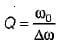and Q is high therefore, the resonant frequency ω0 will be high. Hence, the amplitude response will have a peak always

Test: Frequency Response & Resonance - Question 2

### A network function contains only poles whose real-parts are zero or negative. The network is

Detailed Solution for Test: Frequency Response & Resonance - Question 2

Poles are at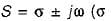can be zero or negative). The system will be stable if the poles at jω-axis are simple.

Test: Frequency Response & Resonance - Question 3

### The minimum amount of hardware required to make a high pass filter is

Detailed Solution for Test: Frequency Response & Resonance - Question 3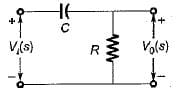Figure above shows a high-pass filter having a transfer function,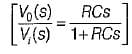Test: Frequency Response & Resonance - Question 4

The Q-factor of a series RLC circuit having R = 10 Ω , L = 0.01 H and C = 100 μF is

Detailed Solution for Test: Frequency Response & Resonance - Question 4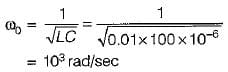Also, bandwidth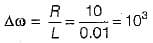∴ Quantity factor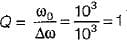Test: Frequency Response & Resonance - Question 5

The input frequency of the given circuit for unity power factor operation is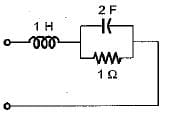Detailed Solution for Test: Frequency Response & Resonance - Question 5

Input impedance,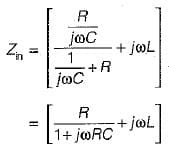or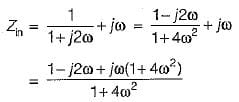For unity p.f. operation, Img(Zin) = 0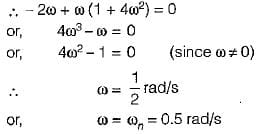Test: Frequency Response & Resonance - Question 6

When XL is equal to Xc , then

Test: Frequency Response & Resonance - Question 7

If the quality factor of a series resonant circuit is increased then, its selectivity will

Detailed Solution for Test: Frequency Response & Resonance - Question 7

Q∝  selectivity

Test: Frequency Response & Resonance - Question 8

The steady state transfer function H(Jω) of the network shown below is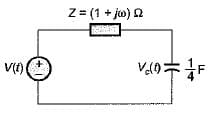Detailed Solution for Test: Frequency Response & Resonance - Question 8

Transfer function,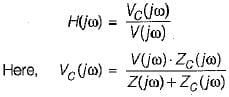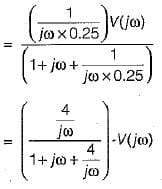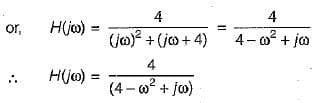Test: Frequency Response & Resonance - Question 9

The transfer function of the circuit shown below is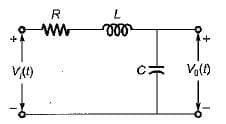Detailed Solution for Test: Frequency Response & Resonance - Question 9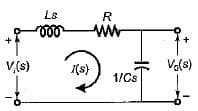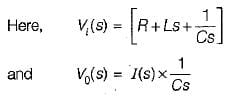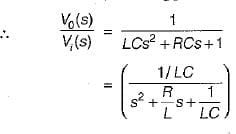Test: Frequency Response & Resonance - Question 10

The figure of merit for a capacitor in parallel with a resistance R is given by

Detailed Solution for Test: Frequency Response & Resonance - Question 10

Figure of merit = Q-factor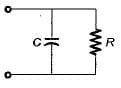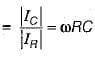## Topicwise Question Bank for Electronics Engineering

101 tests
 Use Code STAYHOME200 and get INR 200 additional OFF Use Coupon Code
Information about Test: Frequency Response & Resonance Page
In this test you can find the Exam questions for Test: Frequency Response & Resonance solved & explained in the simplest way possible. Besides giving Questions and answers for Test: Frequency Response & Resonance, EduRev gives you an ample number of Online tests for practice

101 tests